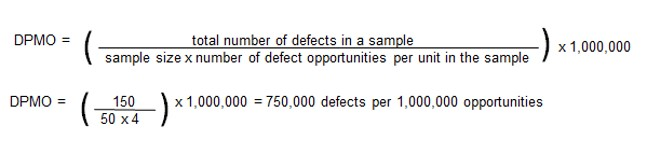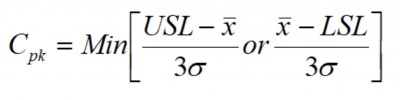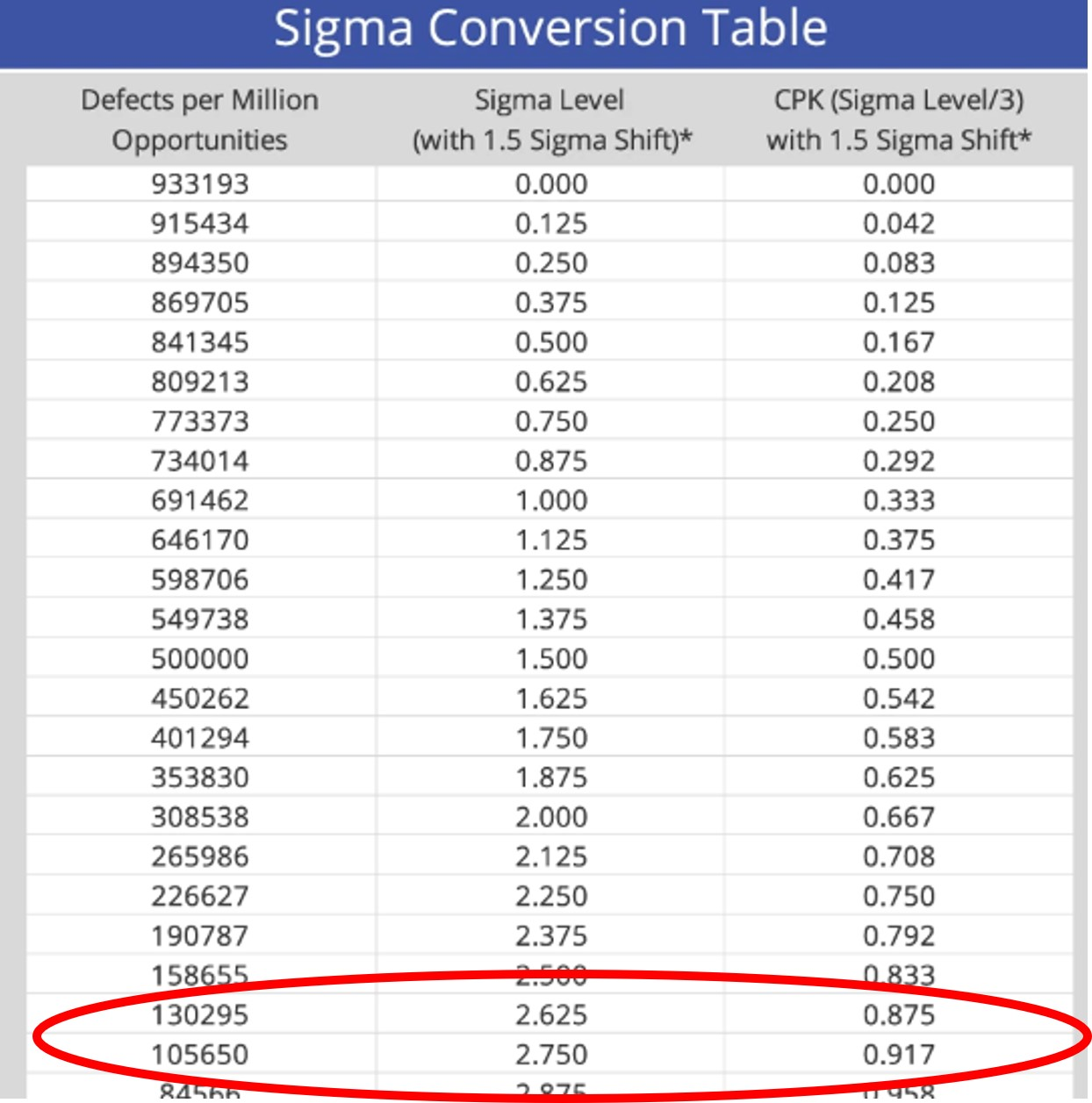There are several metrics you can use to measure how well your process is performing. Let’s look at three of them and discuss how they are calculated and related. The three are DPMO, Sigma Level and Cpk.

## Convert DPMO/Sigma to Cpk

Before discussing how you can convert DPMO (defects per million opportunities) to sigma level and then to a value for Cpk, you must understand what each metric means.

### DPMO

Let’s use an example to illustrate how you would compute DPMO. Assuming you took a sample of 50 documents, totaled the count of all defects found in those 50 documents (assume a total of 150 defects), and then divided that number by your sample size of 50 to get an average estimate of 3 defects per unit or document.To calculate DPMO, you would use this formula:If your organization does not produce a large volume of output, you can also calculate defects per thousand opportunities, or defects per hundred opportunities by multiplying by the appropriate number.

### Sigma Level

Sigma level is a statistical calculation that takes short-term information regarding the defects per million opportunities (DPMO) of a process, factors in the tendency of a process sigma to shift over time (1.5 sigma) and gives a Z score including the 1.5 sigma shift.

### Cpk

Cpk or capability index, is a measure of process capability and indicates the short term ability of your process to meet your customer’s specifications, goals, targets and other numerical expectations. It is calculated as follows where USL/LSL are the upper and lower specification limits and X-bar is the average of the process:## Overview and example: How do you convert DPMO/Sigma to Cpk?

A company, who has recently deployed a Lean Six SIgma effort in their organization, asked their Black Belt (BB) to determine the Cpk of a key process using defect data collected by the quality control department.

The data showed that there were 1,598 total defects found in their monthly production of 13,500 parts. Each part had only one unique possible defect that could occur. The BB’s first calculation was to compute DPMO. This is the BB’s calculation:

DPMO = (Total number of defects / (sample size x number of defect opportunities)) x 1,000,000

DPMO = (1,598/(13,500 x 1)) x 1,000,000 = 1,598/13,500 x 1,000,000 = 118,370

The next step was to use a sigma conversion chart to determine the Sigma Level. As you can see below, the DPMO value falls between a Sigma Level of 2.750 and 2.625.The final step in the process of conversion is to convert the Sigma Level to a Cpk value. According to the table, the estimated Cpk for this process would range from .875-.917. The minimum value of Cpk you should seek to achieve is 1.0 with values of 1.33 or 1.67 being preferred. A Cpk of 2 would be equivalent to a six sigma process.

### 1. Where did the 1.5 sigma shift come from?

The reasoning behind the 1.5 shift developed at Motorola was the realization that processes will deteriorate, drift, or shift in performance over time. This can be caused by changes in raw materials, equipment, people, technology, and other manufacturing changes that occur over time.

After years of data collection and empirical analysis, Motorola consultants Dr. Mikel Harry and Richard Schroeder concluded that the mean of the standard distribution will typically shift about 1.5 sigma.

### 2. How do I use DPMO if my process produces output at a much lower volume?

Instead of calculating Defects Per Million Opportunities and multiplying by 1,000,000, you can adjust your calculations for Defects Per Thousand or Hundred Opportunities and multiply by 1,000 or 100.

### 3. If six sigma is defined as 3.4 DPMO, why does the standard normal table show 3.4 DPMO at 4.5 sigma?

The difference is the inclusion of the 1.5 sigma shift. If you add that to your 4.5 value, you get the 6 sigma value usually defined as 3.4 DPMO.